## Tables index

#### M. S. Peprah, S. A. Kumi

Journal of Geosciences and Geomatics. 2017, 5(3), 96-108 doi:10.12691/jgg-5-3-1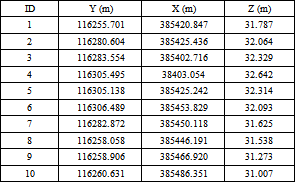• Table 1. Sample of the dataset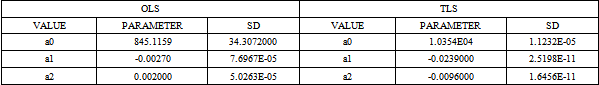• Table 2. Parameters obtained by the OLS and TLS models (Units in metres)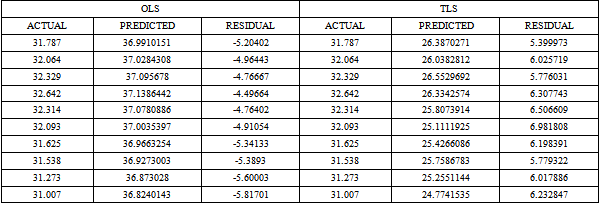• Table 3. Sample results by the OLS and TLS models (Units in metres)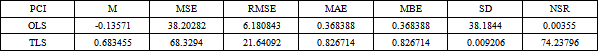• Table 4. Statistical analysis of the OLS and TLS models (Units in metres)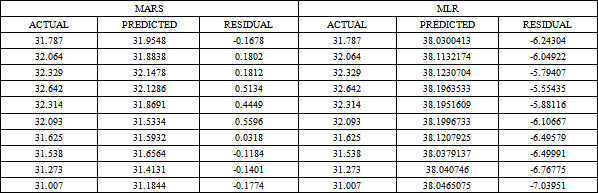• Table 5. Sample results by the MARS and MLR models (Units in metres)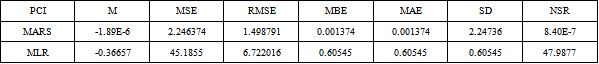• Table 6. Statistical analysis of the MARS and MLR model (Units in metres)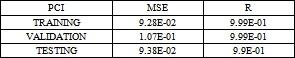• Table 7. ANN optimal results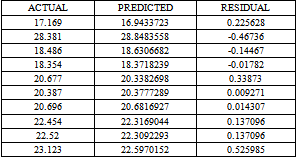• Table 8. Sample results by the ANN model (Units in metres)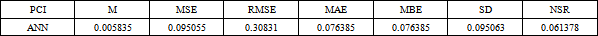• Table 9. Statistical analysis of the ANN model (Units in metres)